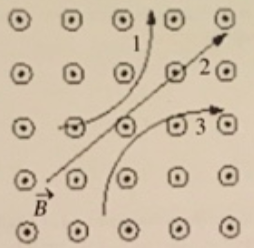# Problem: Three particles travel through a region of space where the magnetic field is out of the page, as shown in the figure. The electric charge of each of the three particles is, respectively.A) 1 is positive, 2 is neutral, and 3 is negativeB) 1 is negative, 2 is neutral, and 3 is positiveC) 1 is neutral, 2 is positive, and 3 is negativeD) 1 is positive, 2 is negative, and 3 is neutralE) 1 is neutral, 2 is negative, and 3 is positive

###### FREE Expert Solution

Right-hand rule for a positive moving charge: Thumb points in the direction of the velocity, fingers point in the direction of the magnetic field, perpendicular to the palm points in the direction of the force.###### Problem Details

Three particles travel through a region of space where the magnetic field is out of the page, as shown in the figure. The electric charge of each of the three particles is, respectively.A) 1 is positive, 2 is neutral, and 3 is negative
B) 1 is negative, 2 is neutral, and 3 is positive
C) 1 is neutral, 2 is positive, and 3 is negative
D) 1 is positive, 2 is negative, and 3 is neutral
E) 1 is neutral, 2 is negative, and 3 is positive